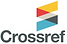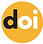top of page## ISSN 2621-5799Published: 30 April 2021

# Middle School Students’ Problem Posing Processes

### Mustafa Zeki Aydoğdu, Elif Türnüklü

Dokuz Eylul University, Turkey10.31014/aior.1993.04.02.227

Pages: 58-67

Keywords: Geometry, Middle School Students, Problem Posing

Abstract

Problem posing has an important place in mathematics education curriculum in many countries. There are a few studies in the literature that reveal students' problem posing processes. The purpose of this research is to examine the problem posing processes of middle school students in geometry. The Research was carried out with 160 students who are in 5th-8th grades (aged 11-14). When the findings obtained in the study were examined, it was understood that the students followed some certain ways while posing problems, identified in the research. The processes followed by the students while they are posing problems are classified into 5 steps. It has been observed that the students do not go through all steps in problem posing processes; while some students use all of these steps, the others use some of them. The students are divided into four different types according to the way they used in their problem posing processes.

References

1. Abu-Elwan, R. (2011). Effect of using Cabri II environment by prospective teachers on fractal geometry problem posing. In M. Joubert, A. Clark-Wilson & M. McCabe (Eds.), Proceedings of the 10th International Conference for Technology in Mathematics Teaching (pp. 56-61). Portsmouth, UK.

2. Adelina, R. and Fatma, M. (2018). Enhancing students’ mathematical problem posing skill through writing in performance tasks strategy. In Journal of Physics: Conference Series (Vol. 948, No. 1, p. 012022). IOP Publishing.

3. Brown, S. I. and Walter, M. I. (2005). The art of problem posing third edition. London: LEA.

4. Büyüköztürk, Ş., Kılıç Çakmak, E., Akgün, Ö.E., Karadeniz. Ş. and Demirel. F. (2017). Scientific research methods. 17th edition, Ankara: Pegem Akademi.

5. Cai, J. and Hwang, S. (2002). Generalized and generative thinking in US and Chinese students’ mathematical problem solving and problem posing. Journal of Mathematical Behavior, 21, 401-421.

6. Cankoy, O. and Darbaz, S. (2010). The effect of problem posing based problem solving education on the success of understanding a problem. Hacettepe University Faculty of Education Journal, 38, 11-24.

7. Christou, C., Mousoulides, N., Pittalis, M. and Pitta-Pantazi, D. (2005). Problem solving and problem posing in a dynamic geometry environment. The Mathematics Enthusiast, 2(2), 124-143.

8. Crespo, S. (2003). Learning to pose mathematical problems: Exploring changes in preservice teachers’ practices. Educational Studies in Mathematics, 52(3), 243-270.

9. Crespo, S. and Sinclair, N. (2008). What makes a problem mathematically interesting? inviting prospective teachers to pose better problems. Journal of Mathematics Teacher Education, 11(5), 395–415.

10. Cunningham, R. F. (2004). Problem posing: An opportunity for increasing student responsibility. Mathematics and Computer Education, 38(1), 83-39.

11. Ekici, D. (2016). Investigation of middle school students' mathematical problem posing strategies (Master's thesis). Dokuz Eylul University Institute of Educational Sciences, Izmir.

12. English, L. D. (1997a). The development of fifth-grade children’s problem- posing abilities. Educational Studies in Mathematics, 34, 183-217.

13. Geçici, M. E. (2018). Analyzing Eighth Grade Students' Skills of Posing Geometry Problems (Master's Thesis). Dicle University Institute of Educational Sciences, Diyarbakır.

14. Fukuda, C. and Kakihana, K. (2009). Problem posing and its environment with technology. In Proceeding of 33rd conference of Japan Society for Science Education.

15. Işık, C. and Kar, T. (2015). Analyzing the problems that sixth grade students pose for the open-ended verbal story about fractions. Turkish Journal of Computer and Mathematics Education, 6(2), 230-249.

16. Kılıç, Ç. (2013). Determining the performance of the elementary school teacher candidates in different problem posing situations. Journal of Educational Sciences in Theory and Practice, 13(2), 1195-1211.

17. Kojima, K., Miwa, K. and Matsui, T. (2009). Study on support of learning from examples in problem posing as a production task.

19. Leung, S. S., and Silver, E. A. (1997). The role of task format, mathematics knowledge, and creative thinking on the arithmetic problem posing of prospective elementary school teachers. Mathematics Education Research Journal, 9(1), 5-24.

20. Ministry of National Education. (2009). Primary school mathematics lesson 6th - 8th grades' curriculum and guide. Ankara: MEB Printing House.

21. Ministry of National Education. (2013). Middle school mathematics lesson (grades 5, 6, 7 and 8) curriculum. Ankara: MEB Printing House.

22. Ministry of Education. (2018). Mathematics curriculum (primary and middle school 1, 2, 3, 4, 5, 6, 7 and 8th grades).Ankara: MEB Printing House.

23. National Council of Teachers of Mathematics (NCTM). (2000). Principles and standards for school mathematics. Reston, VA: NCTM.

24. Ngah, N., Ismail, Z., Tasir, Z. and Mohamad Said, M. N. H. (2016). Students’ ability in free, semi-structured and structured problem posing situations. Advanced Science Letters, 22(12), 4205-4208.

25. Osana, H.P. and Pelczer, İ. (2015). A review on problem posing in teacher education. jinfa cai and james middleton (seri ed.), Matematical Problem Posing From Research to Effective Practice. 469-490.

26. Pelczer, I. and Gamboa, F. (2009). Problem posing: Comparison between experts and novices. Tzekaki, M., Kaldrimidou, & C.Sakonidis (Eds.), Proceedings of the 33rd International Conference of the International Group for the Psychology Mathematics Education, (pp. 353-360). Thessaloniki, Greece: PME.

27. Rosli, R., Mary, M.C., Goldsby, D., Gonzales, E., Onwuegbuzie, A. J. and Capraro C. M. (2015). Middle-grade preservice teachers’ mathematical problem solving and problem posing. jinfa cai and james middleton (seri ed.), Mathematical Problem Posing From Research to Effective Practice, 333-355.

28. Silver, E. A. (1994). On mathematical problem posing. For the Learning of Mathematics, 14(1), 19-28.

29. Silver, E. A. and Cai, J. (1996). An analysis of arithmetic problem posing by middle school students. Journal for Research in Mathematics Education, 27, 521–539.

30. Singer, F. M., Voica, C. and Pelczer, I. (2017). Cognitive styles in posing geometry problems: Implications for assessment of mathematical creativity. ZDM Mathematics Education, 49(1), 37–52.

31. https://doi.org/10.1007/s11858-016-0820-x.

32. Yaman, S. and Dede, Y. (2005). Problem posing applications in mathematics and science education. Ondokuz Mayıs University Faculty of Education Journal, 20, 1-11.

33. Van Harpen, X. Y. and Presmeg, N. (2013). An investigation of relationships between students' mathematical problem-posing abilities and their mathematical content knowledge. Educational Studies in Mathematics, 83(1), 117-132.

bottom of page